# ISRO Scientist or Engineer Electrical 2015

Instructions

For the following questions answer them individually

Question 11

# The Burden ofprotective Current Transformer (CT) is specified inQuestion 12

# In the network shown in the figure below, the current in the 12 Ohm resistor is 5 Amps, the battery voltage E is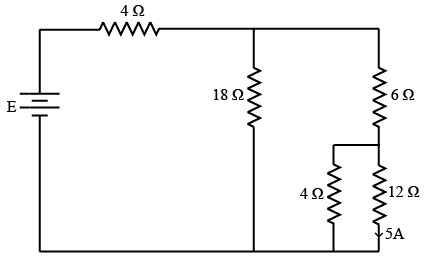Question 13

# What is the equivalent capacitance of the following circuit?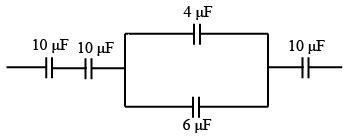Question 14

# The relation between the flux density (B) and Magnetic field intensity (H) is given by $$B = \mu H$$. What is $$\mu$$ in this relation?Question 15

# Powersupply to a 10 pole induction motor is supplied by 4 pole alternator which is driven at 1500 RPM. If the motor runs with slip of 4%, whatis its speed?Question 16

# A power factor meter connected in a circuit indicates pf of 0.6 lagging. To improve the power factor, we have to insert the following componentin the circuit.Question 17

# The logic evaluated by the circuit at the output is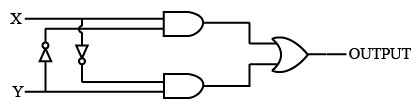Question 18

# The figure shows a half wave rectifier circuit with input voltage $$U(t) = 10 \sin (100 \pi t)$$ volts. Assuming ideal diode characteristics with zero forward voltage drop and zero reverse current, the average power consumed in watts by the load resistance RL is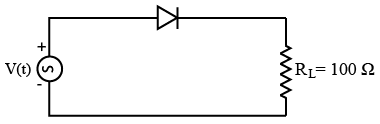Question 19

# The truth table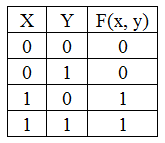Represent the Boolean functionQuestion 20

# A three stage Johnson counter ring in figure is clocked at a constant frequency of $$f_c$$. from starting state of $$Q_0, Q_1, Q_2 = 101$$. The frequencyof output $$Q_0, Q_1, Q_2,$$ will be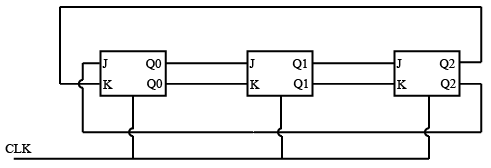OR